# Quiz 25: Genetics Basis of Cancer

Biology

Height, weight, pigmentation in flowers and skin, milk production metabolic rate, speed and wavy hair as well as hidden traits such as musical talent, blood pressure, longevity and complex diseases like cancer, diabetes, hypertension and arthritis are some examples for quantitative traits. These are so called because their expression in individual depends on their mutual expression in various amounts. The quantitative traits can be described by statistical study of traits that is calculating Mean, Variance (V), Standard Deviation (S.D) and degree of variation for all measured values of individuals in a population for a trait. These statistical tools help us in analysing the variation of quantitative traits with in a group of individuals.

(A)First, we have to calculate the means of height and weights of the students and difference between each value and mean.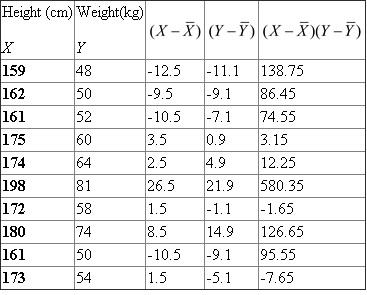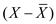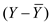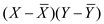Calculate variance and standard deviation ( S.D ) for both height and weight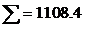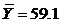Variance of height V X = 140.277 Standard deviation of height ( S.D X ) =11.84 Variance of weight V Y =121.43 Standard deviation of weight ( S.D Y ) =11.02 Then calculate covariance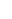Covariance CoV (X,Y) =123.15 By using above data let's calculate correlation coefficient( r )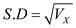(B)It is statistically significant with 8 degrees of freedom. That mean the association of these two variables is not because of random sampling errors. The association is true and frequent.

Heritability is important in agriculture because, in selective breeding heritability plays a major role. The breeder selects the plants as breeding stock for next generation, whose heritability value is high to improve the desired traits. People misunderstood the concept of heritability, by thinking that the variation of a trait is governs by genetics alone. But, Heritability is the amount of phenotypic variation is due to genetic variation in a particular population raised in a particular environment. Heritability depends on both genetical and environmental factors. Heritability does not describe the relative impact of the genetic variation and effect of environment on the outcome of a trait.